# Posts tagged as “combination”

Given a list of words, list of  single letters (might be repeating) and score of every character.

Return the maximum score of any valid set of words formed by using the given letters (words[i] cannot be used two or more times).

It is not necessary to use all characters in letters and each letter can only be used once. Score of letters 'a''b''c', … ,'z' is given by scorescore, … , score respectively.

Example 1:

Input: words = ["dog","cat","dad","good"], letters = ["a","a","c","d","d","d","g","o","o"], score = [1,0,9,5,0,0,3,0,0,0,0,0,0,0,2,0,0,0,0,0,0,0,0,0,0,0]
Output: 23
Explanation:
Score  a=1, c=9, d=5, g=3, o=2
Given letters, we can form the words "dad" (5+1+5) and "good" (3+2+2+5) with a score of 23.
Words "dad" and "dog" only get a score of 21.

Example 2:

Input: words = ["xxxz","ax","bx","cx"], letters = ["z","a","b","c","x","x","x"], score = [4,4,4,0,0,0,0,0,0,0,0,0,0,0,0,0,0,0,0,0,0,0,0,5,0,10]
Output: 27
Explanation:
Score  a=4, b=4, c=4, x=5, z=10
Given letters, we can form the words "ax" (4+5), "bx" (4+5) and "cx" (4+5) with a score of 27.
Word "xxxz" only get a score of 25.

Example 3:

Input: words = ["leetcode"], letters = ["l","e","t","c","o","d"], score = [0,0,1,1,1,0,0,0,0,0,0,1,0,0,1,0,0,0,0,1,0,0,0,0,0,0]
Output: 0
Explanation:
Letter "e" can only be used once.

Constraints:

• 1 <= words.length <= 14
• 1 <= words[i].length <= 15
• 1 <= letters.length <= 100
• letters[i].length == 1
• score.length == 26
• 0 <= score[i] <= 10
• words[i]letters[i] contains only lower case English letters.

## Solution: Combination

Time complexity: O(2^n)
Space complexity: O(n)

## C++

Given an array of strings arr. String s is a concatenation of a sub-sequence of arr which have unique characters.

Return the maximum possible length of s.

Example 1:

Input: arr = ["un","iq","ue"]
Output: 4
Explanation: All possible concatenations are "","un","iq","ue","uniq" and "ique".
Maximum length is 4.


Example 2:

Input: arr = ["cha","r","act","ers"]
Output: 6
Explanation: Possible solutions are "chaers" and "acters".


Example 3:

Input: arr = ["abcdefghijklmnopqrstuvwxyz"]
Output: 26


Constraints:

• 1 <= arr.length <= 16
• 1 <= arr[i].length <= 26
• arr[i] contains only lower case English letters.

## Solution: Combination + Bit

Time complexity: O(2^n)
Space complexity: O(n)

## C++

Solution 2: DP

Time complexity: O(2^n)
Space complexity: O(2^n)

## C++

Given two integers n and k, return all possible combinations of k numbers out of 1 … n.

Example:

Input: n = 4, k = 2
Output:
[
[2,4],
[3,4],
[2,3],
[1,2],
[1,3],
[1,4],
]

## Solution: DFS

Time complexity: O(C(n, k))
Space complexity: O(k)

## Related Problems

Given a set of distinct integers, nums, return all possible subsets (the power set).

Note: The solution set must not contain duplicate subsets.

Example:

Input: nums = [1,2,3]Output:[  ,  ,  ,  [1,2,3],  [1,3],  [2,3],  [1,2],  []]

# Solution: Combination

Time complexity: O(2^n)
Space complexity: O(n)

# 0-1 Knapsack Problem 0-1背包问题

## Problem

Given N items, w[i] is the weight of the i-th item and v[i] is value of the i-th item. Given a knapsack with capacity W. Maximize the total value. Each item can be use 0 or 1 time.

0-1背包问题的通常定义是：一共有N件物品，第i件物品的重量为w[i]，价值为v[i]。在总重量不超过背包承载上限W的情况下，能够获得的最大价值是多少？每件物品可以使用0次或者1次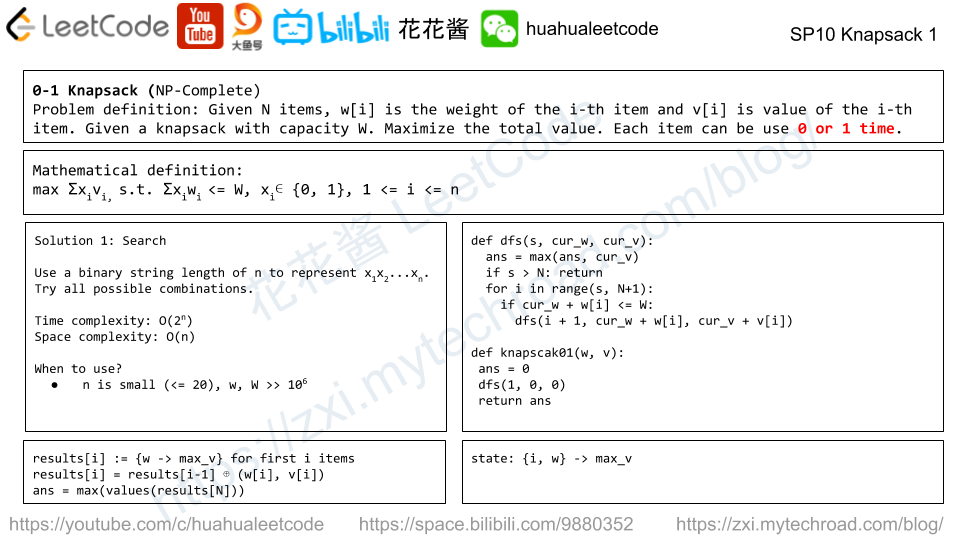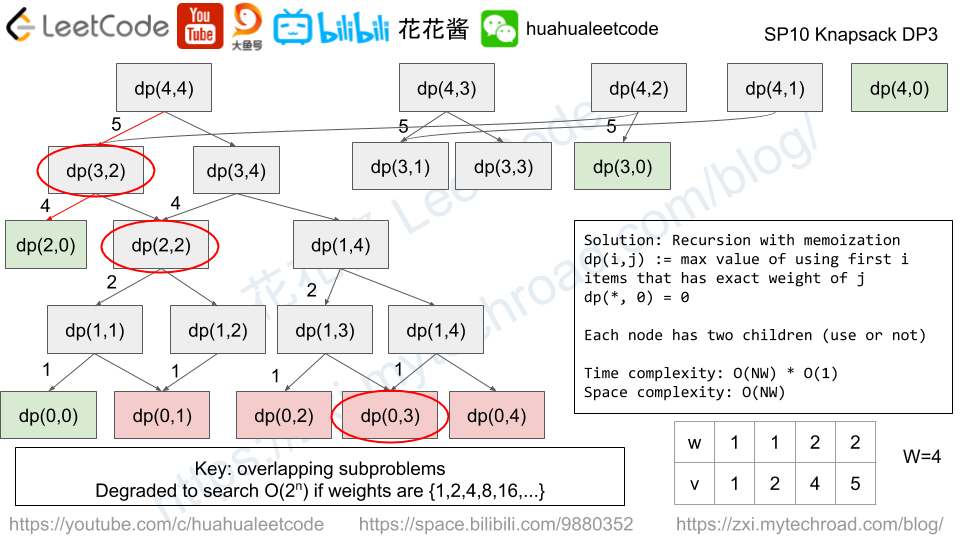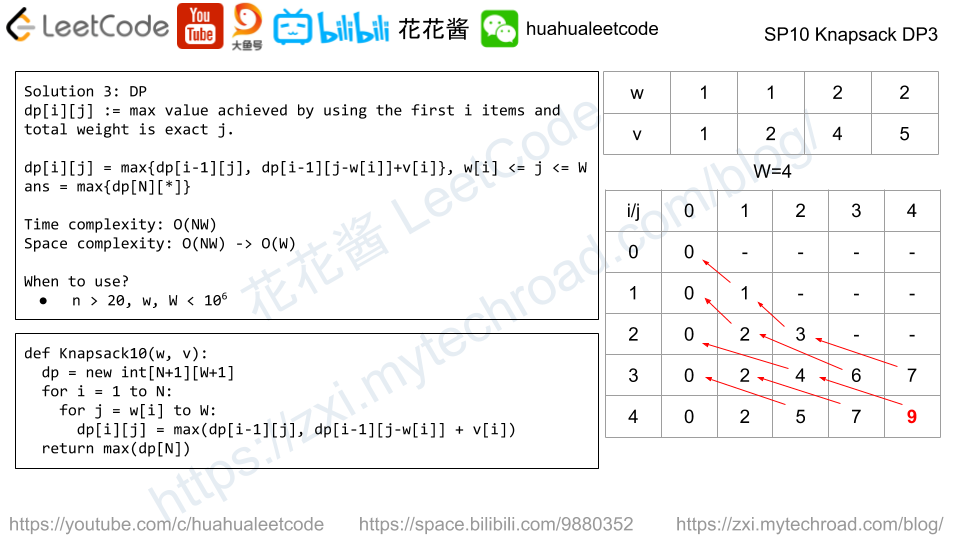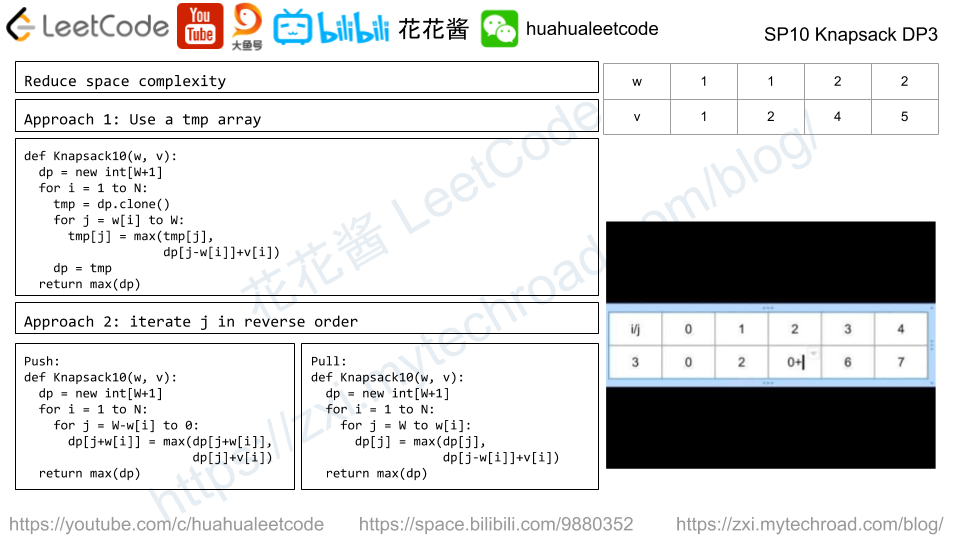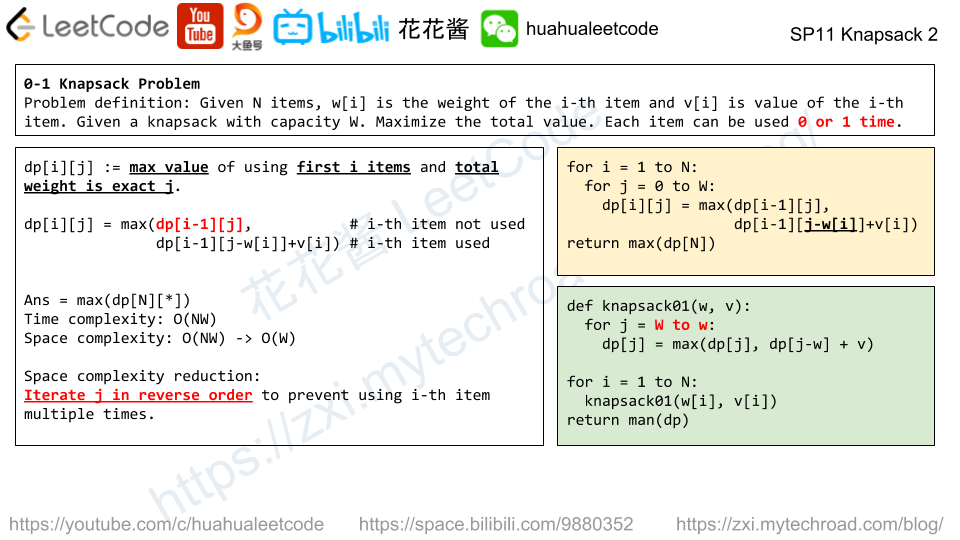# Unbounded Knapsack Problem 完全背包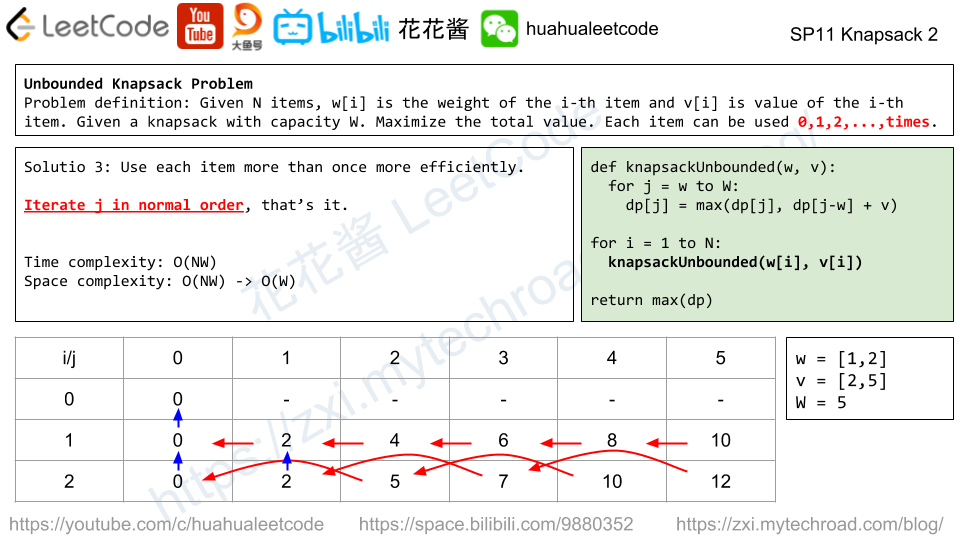# Bounded Knapsack Problem 多重背包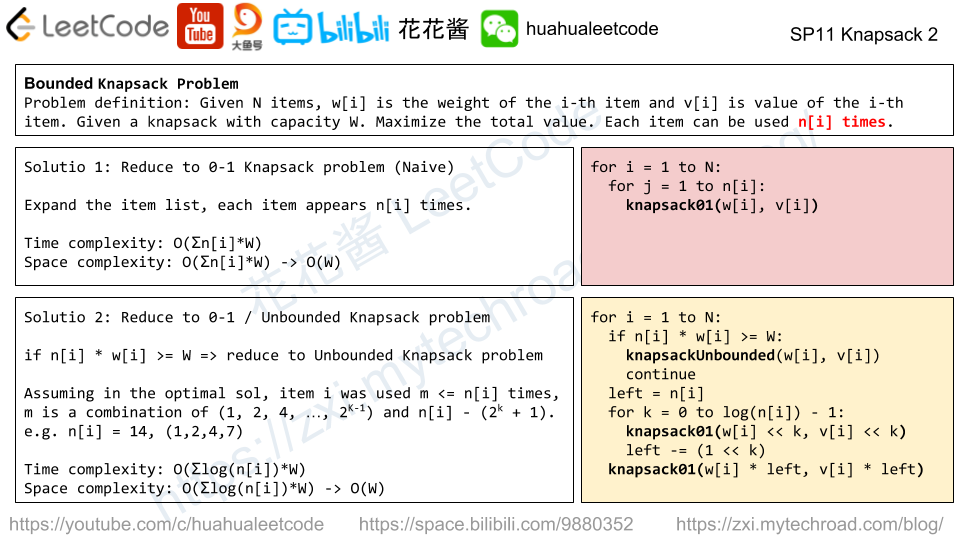Mission News Theme by Compete Themes.